# AP Calculus BC Practice Test 11

### Test Information10 questions20 minutes

Calculator Disallowed

1. If y = tan(2πx), then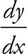= ?

2.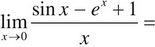3.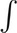tan2 x dx

4. For 0 ≤ t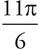, an object travels along the ellipse given by x = 2sin t, y = -3cos t. At t =, the object leaves the ellipse and travels along the line tangent to the path at that point. What is the slope of the tangent line at t =?

5. If f(x) =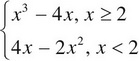, which of the following is true?

6.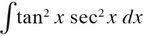7. The position of a particle at time t ≥ 0, in seconds, is given by x = 2t3 + 3t2 – 12t and y = 2t3 – 15t2 + 24t. At what values of t is the particle at rest?

8.3x csc2 x2 dx =

9. If f(x) = log5(x3 - x - 1), then f′(2) =

10. What is the value of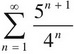?